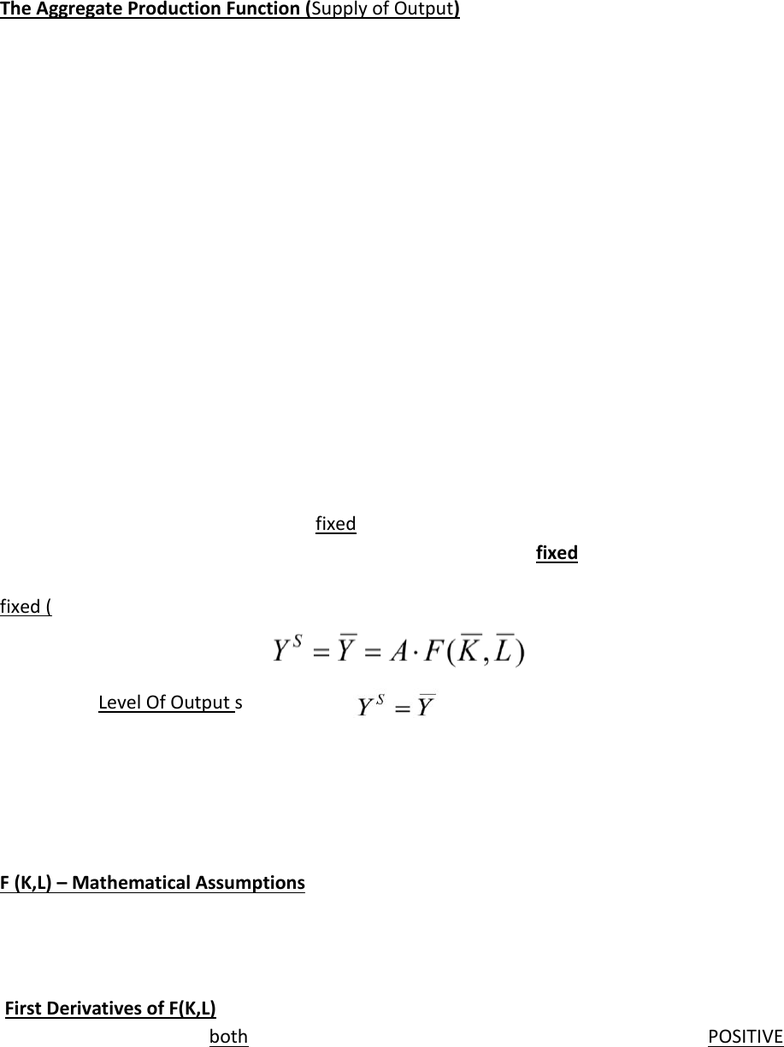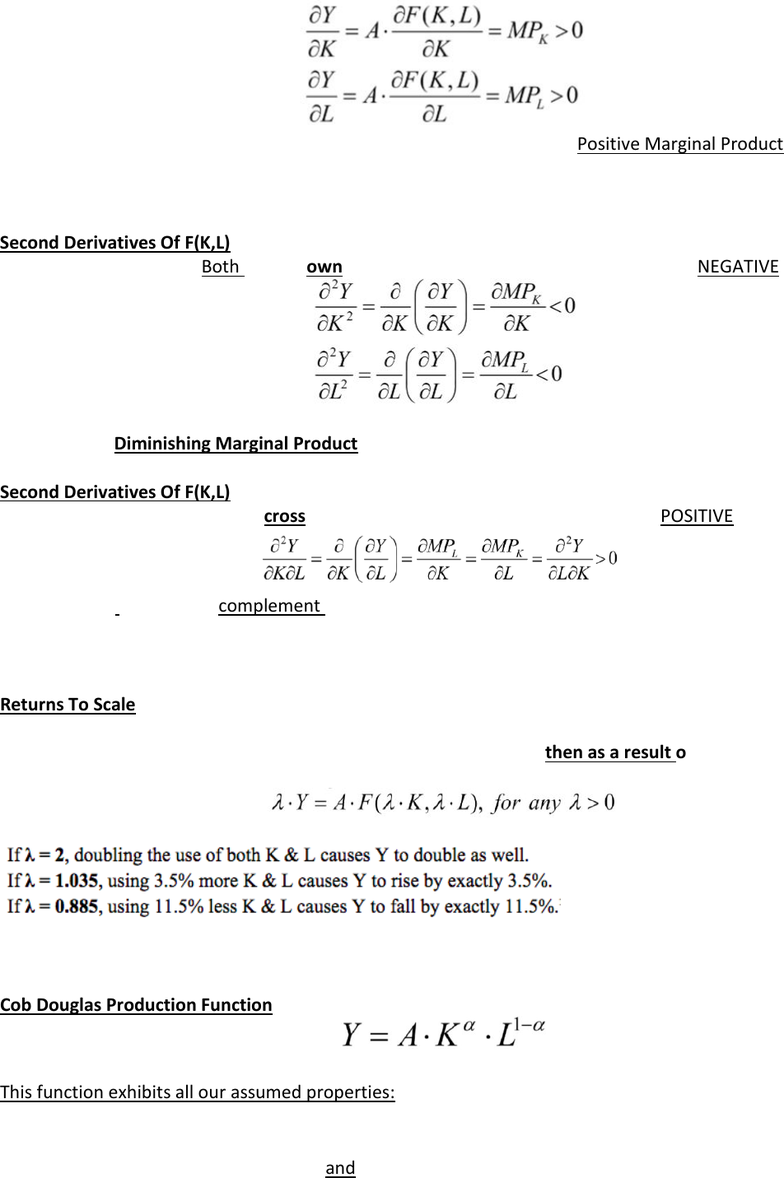# MGEB06H3 Lecture 3: Chapter 3 notes

22 views7 pagesChapter 3- The Classical Model Of The Closed Economy In the Long Run
The Aggregate Production Function (Supply of Output)
Y= A F ( K, L)
K= The aggregate real stock of productive capital
L= the aggregate real stock (or supply) of labour
F ( ) = is a productive function that transforms inputs (K&L) into real output (Y= real GDP)
A= picks up the impact of all left out inputs and technological features.
Residual collecting up all these left out terms so it has been called Solow Residual
Another common name is Total Factor Productivity since it picks up productivity of all inputs in total
The Term A can change when:
o There is a change in energy prices
o There is a change in weather
o There is a change in rules & regulations
o There is a change in management practices
o There is a change in technology
Taxes(T), Government spending on goods & services (G), the nominal Money Supply (M), and many other
economic variables have no direct bearing or influence on the level of Y in the LR
In the Long Run:
Prices are perfectly flexible
Capital & technology are both fixed
K = K , A & F( ) are all fixed
If we also assume that the supply of labour is also fixed, L = L, then as a result the supply of output is also
fixed (in the long run)
We call the Level Of Output such that:
The natural rate level of output
The full employment level of output
The level of Potential output
The Long- run equilibrium level of output
The non-accelerating inflation rate level of output
F (K,L) Mathematical Assumptions
1. Signs of the first partial Deviates
2. Signs of the second own & cross partial derivatives
3. Impacts of equi-proportionate changes in all inputs- Returns To Scale- what return to output is
experienced if we scale all inputs up or down by the same percentage
First Derivatives of F(K,L)
Assume that the signs of both first partial derivatives of the production function are POSITIVE
Unlock document

This preview shows pages 1-2 of the document.
Unlock all 7 pages and 3 million more documents.Assuming that both inputs are always useful inputs as they always have Positive Marginal Product
MPL = Marginal Product Of Labour
MPk = Marginal Product Of Capital
Second Derivatives Of F(K,L)
Assume that the signs of Both second own partial derivative of the prod. Function are NEGATIVE
Assuming that both inputs contribute less and less additional output as their use in increased. That is, both
inputs exhibit Diminishing Marginal Product
Second Derivatives Of F(K,L)
Assume that the signs of second cross partial derivative of the prod. Function are POSITIVE
Assuming that both inputs complement each other in the production process
A little bit more (less) K causes the Marginal Product of Labour to rise (fall)
A little bit more (less) L causes the Marginal Product Of Capital to rise (fall)
Returns To Scale
Assume the production function exhibits Constant Returns To Scale (CRTS)
If all inputs (K&L) are scaled up (or down) by the same percentage then as a result output moves up (or
down) by the exact same percentage
For ex:
If the equation holds then CRTS has been established
Cob Douglas Production Function
Where 0 < a < 1
This function exhibits all our assumed properties:
Positive Marginal Products (of L&K)
Diminishing Marginal Products (for L&K)
Complementarity between L & K and CRTS
Unlock document

This preview shows pages 1-2 of the document.
Unlock all 7 pages and 3 million more documents.

# Get access

\$10 USD/m
Billed \$120 USD annually
Homework Help
Class Notes
Textbook Notes